# Quantum physics quantum theory quantum mechanics Part 2

• Slides: 60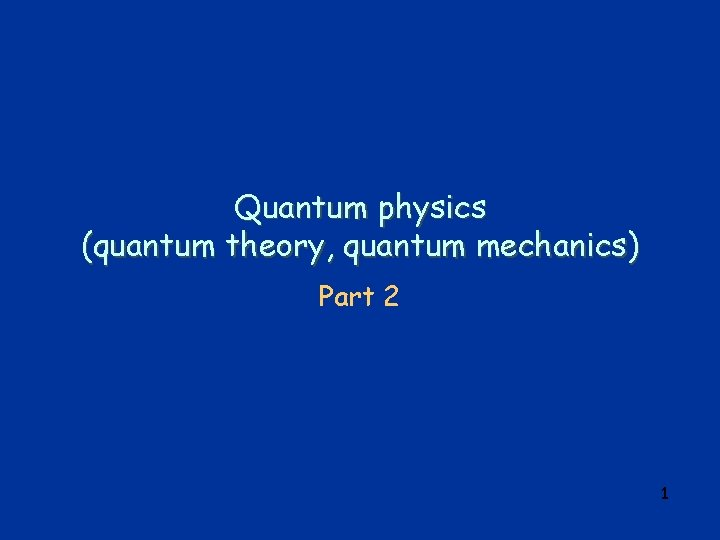Quantum physics (quantum theory, quantum mechanics) Part 2 1Summary of 1 st lecture q classical physics explanation of black-body radiation failed q Planck’s ad-hoc assumption of “energy quanta” of energy Equantum = h , leads to a radiation spectrum which agrees with experiment. q old generally accepted principle of “natura non facit saltus” violated q Opens path to further developments 2Outline q Introduction q cathode rays …. electrons q photoelectric effect l observation l studies l Einstein’s explanation q models of the atom q Summary 3Electron q Cathode rays: l During 2 nd half of 19 th century, many physicists do experiments with “discharge tubes”, i. e. evacuated glass tubes with “electrodes” at ends, electric field between them (HV) l 1869: discharge mediated by rays emitted from negative electrode (“cathode”) l rays called “cathode rays” l study of cathode rays by many physicists – find o o cathode rays appear to be particles cast shadow of opaque body deflected by magnetic field negative charge l eventually realized cathode rays were particles – named them electrons 4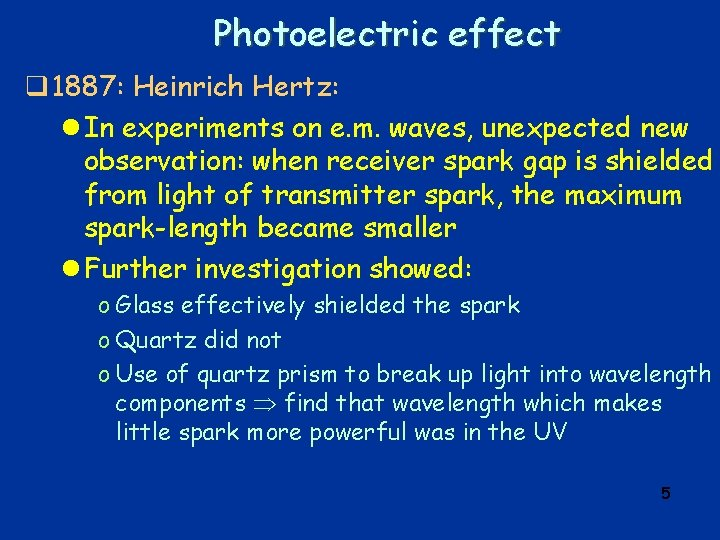Photoelectric effect q 1887: Heinrich Hertz: l In experiments on e. m. waves, unexpected new observation: when receiver spark gap is shielded from light of transmitter spark, the maximum spark-length became smaller l Further investigation showed: o Glass effectively shielded the spark o Quartz did not o Use of quartz prism to break up light into wavelength components find that wavelength which makes little spark more powerful was in the UV 5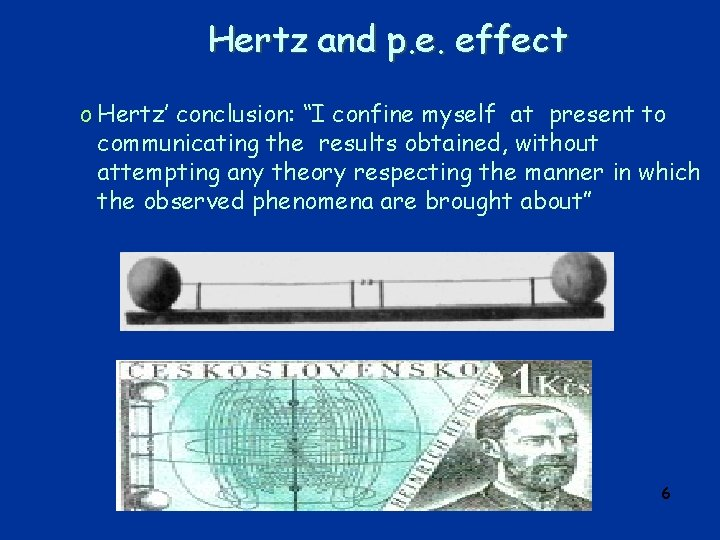Hertz and p. e. effect o Hertz’ conclusion: “I confine myself at present to communicating the results obtained, without attempting any theory respecting the manner in which the observed phenomena are brought about” 6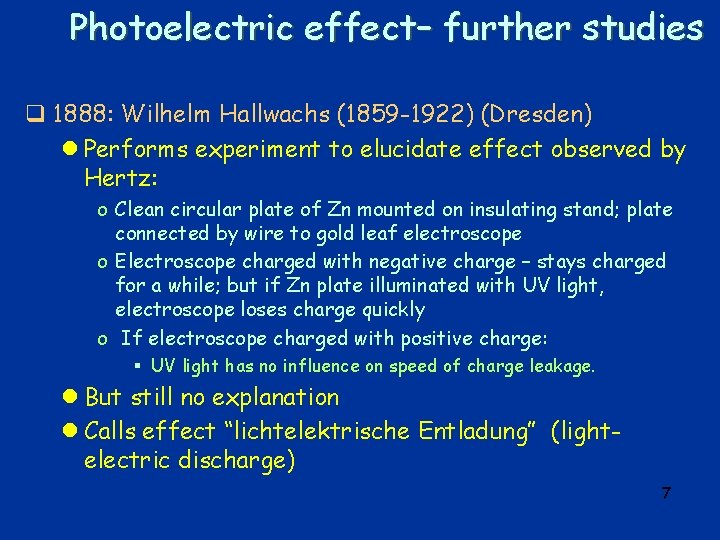Photoelectric effect– further studies q 1888: Wilhelm Hallwachs (1859 -1922) (Dresden) l Performs experiment to elucidate effect observed by Hertz: o Clean circular plate of Zn mounted on insulating stand; plate connected by wire to gold leaf electroscope o Electroscope charged with negative charge – stays charged for a while; but if Zn plate illuminated with UV light, electroscope loses charge quickly o If electroscope charged with positive charge: § UV light has no influence on speed of charge leakage. l But still no explanation l Calls effect “lichtelektrische Entladung” (lightelectric discharge) 7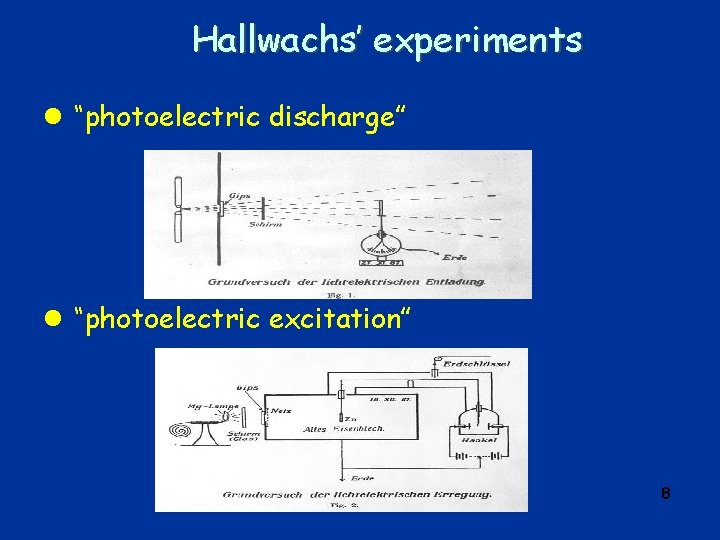Hallwachs’ experiments l “photoelectric discharge” l “photoelectric excitation” 8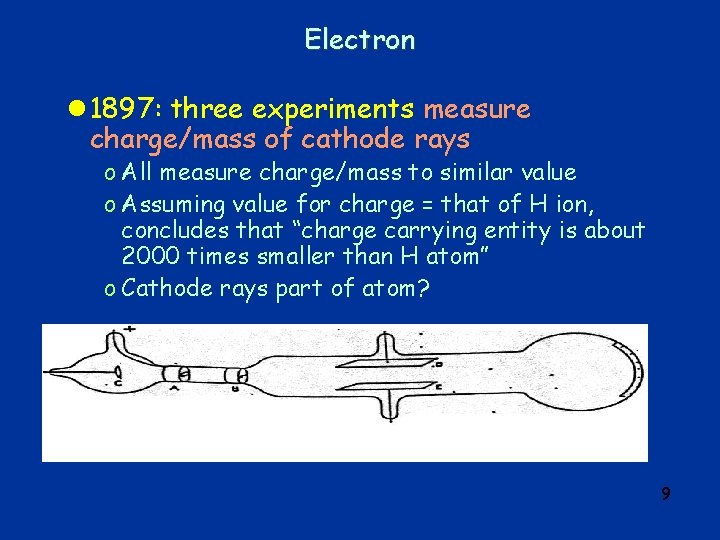Electron l 1897: three experiments measure charge/mass of cathode rays o All measure charge/mass to similar value o Assuming value for charge = that of H ion, concludes that “charge carrying entity is about 2000 times smaller than H atom” o Cathode rays part of atom? 9Further studies of photoelectric effect q 1899: J. J. Thomson: studies of photoelectric effect: l Modifies cathode ray tube: make metal surface to be exposed to light the cathode in a cathode ray tube l Finds that particles emitted due to light are the same as cathode rays (same e/m) 10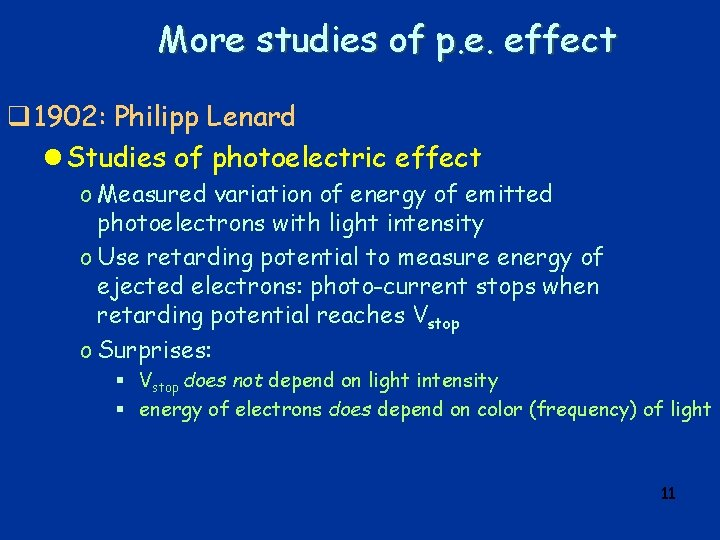More studies of p. e. effect q 1902: Philipp Lenard l Studies of photoelectric effect o Measured variation of energy of emitted photoelectrons with light intensity o Use retarding potential to measure energy of ejected electrons: photo-current stops when retarding potential reaches Vstop o Surprises: § Vstop does not depend on light intensity § energy of electrons does depend on color (frequency) of light 11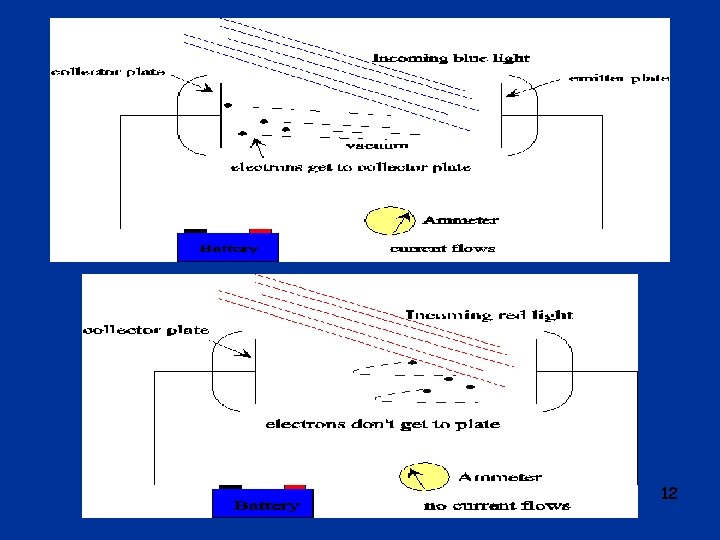12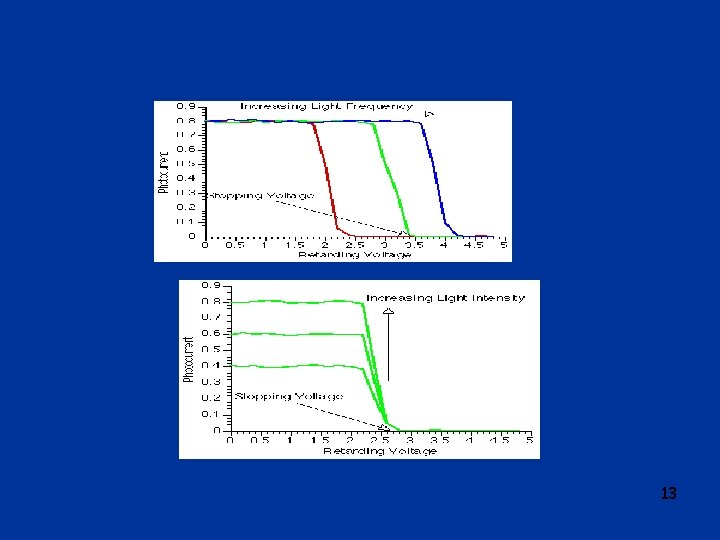13Explanation of photoelectric effect q 1905: Albert Einstein (1879 -1955) (Bern) l Gives explanation of observation relating to photoelectric effect: o Assume that incoming radiation consists of “light quanta” of energy h (h = Planck’s constant, =frequency) o electrons will leave surface of metal with energy E=h –W W = “work function” = energy necessary to get electron out of the metal o there is a minimum light frequency for a given metal, that for which quantum of energy is equal to work function o When cranking up retarding voltage until current stops, the highest energy electrons must have had energy e. Vstop on leaving the cathode o Therefore e. Vstop = h – W 14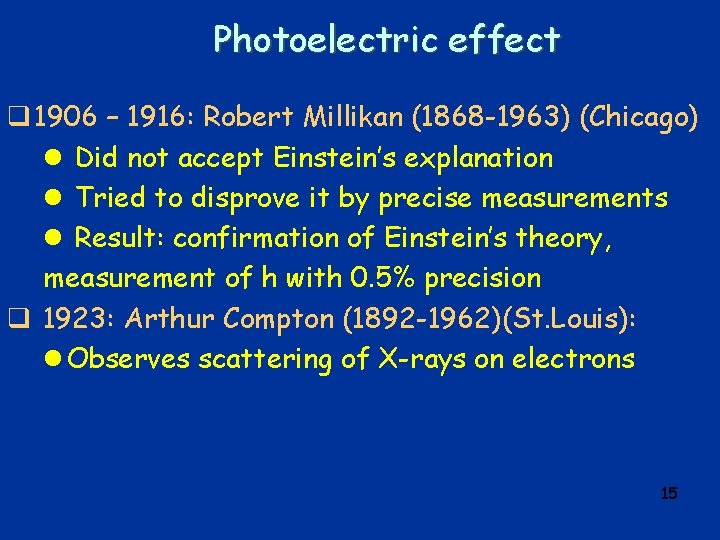Photoelectric effect q 1906 – 1916: Robert Millikan (1868 -1963) (Chicago) l Did not accept Einstein’s explanation l Tried to disprove it by precise measurements l Result: confirmation of Einstein’s theory, measurement of h with 0. 5% precision q 1923: Arthur Compton (1892 -1962)(St. Louis): l Observes scattering of X-rays on electrons 15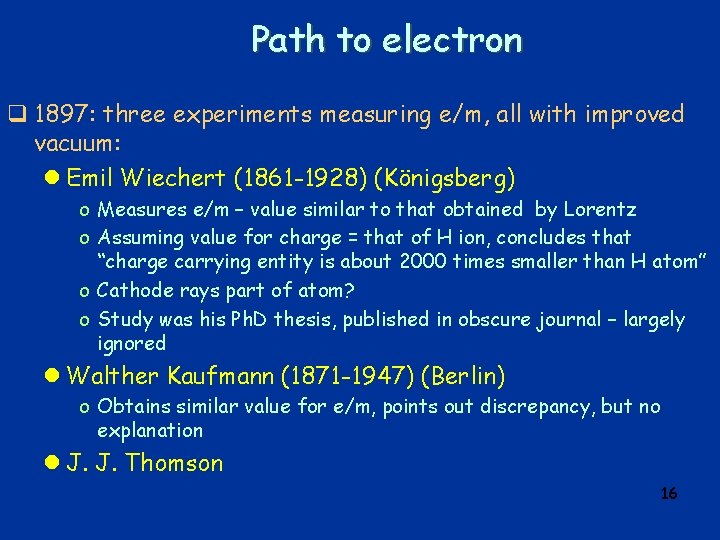Path to electron q 1897: three experiments measuring e/m, all with improved vacuum: l Emil Wiechert (1861 -1928) (Königsberg) o Measures e/m – value similar to that obtained by Lorentz o Assuming value for charge = that of H ion, concludes that “charge carrying entity is about 2000 times smaller than H atom” o Cathode rays part of atom? o Study was his Ph. D thesis, published in obscure journal – largely ignored l Walther Kaufmann (1871 -1947) (Berlin) o Obtains similar value for e/m, points out discrepancy, but no explanation l J. J. Thomson 16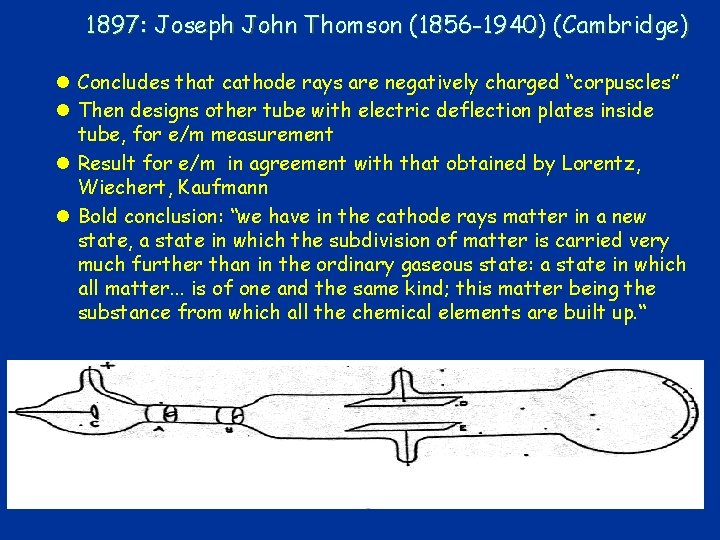1897: Joseph John Thomson (1856 -1940) (Cambridge) l Concludes that cathode rays are negatively charged “corpuscles” l Then designs other tube with electric deflection plates inside tube, for e/m measurement l Result for e/m in agreement with that obtained by Lorentz, Wiechert, Kaufmann l Bold conclusion: “we have in the cathode rays matter in a new state, a state in which the subdivision of matter is carried very much further than in the ordinary gaseous state: a state in which all matter. . . is of one and the same kind; this matter being the substance from which all the chemical elements are built up. “ 17WHY CAN'T WE SEE ATOMS? q “seeing an object” l = detecting light that has been reflected off the object's surface l light = electromagnetic wave; l “visible light”= those electromagnetic waves that our eyes can detect l “wavelength” of e. m. wave (distance between two successive crests) determines “color” of light l wave hardly influenced by object if size of object is much smaller than wavelength l wavelength of visible light: between 4 10 -7 m (violet) and 7 10 -7 m (red); l diameter of atoms: 10 -10 m q generalize meaning of seeing: l seeing is to detect effect due to the presence of an object l quantum theory “particle waves”, with wavelength 1/(m v) l use accelerated (charged) particles as probe, can “tune” wavelength by choosing mass m and changing velocity v l this method is used in electron microscope, as well as in “scattering 18 experiments” in nuclear and particle physicsWHAT IS INSIDE AN ATOM? q THOMSON'S MODEL OF ATOM l (“RAISIN CAKE MODEL”): o atom = sphere of positive charge (diameter 10 -10 m), o with electrons embedded in it, evenly distributed (like raisins in cake) q Geiger & Marsden’s SCATTERING EXPERIMENT: l (Geiger, Marsden, 1906 - 1911) (interpreted by Rutherford, 1911) l get particles from radioactive source l make “beam” of particles using “collimators” (lead plates with holes in them, holes aligned in straight line) l bombard foils of gold, silver, copper with beam l measure scattering angles of particles with scintillating screen (Zn. S). 19Models of Atom q. J. J. Thomson’s model: l“Plum pudding or raisin cake model” o atom = sphere of positive charge (diameter 10 -10 m), o with electrons embedded in it, evenly distributed (like raisins in cake) o i. e. electrons are part of atom, can be kicked out of it – atom no longer indivisible! 20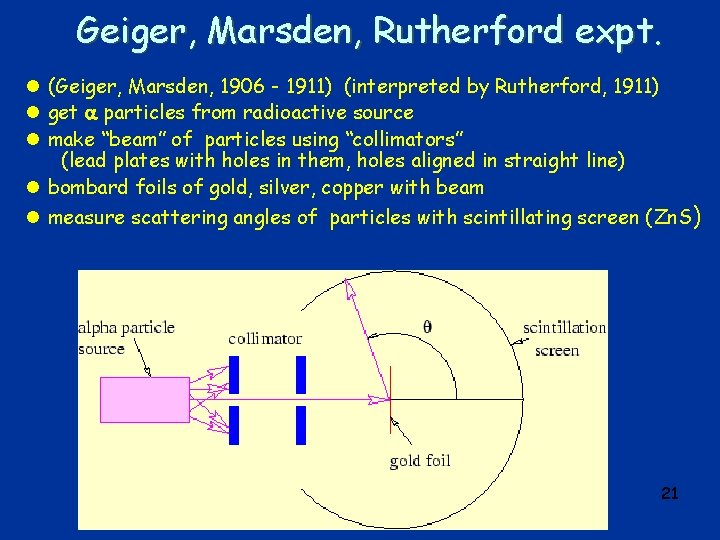Geiger, Marsden, Rutherford expt. l (Geiger, Marsden, 1906 - 1911) (interpreted by Rutherford, 1911) l get particles from radioactive source l make “beam” of particles using “collimators” (lead plates with holes in them, holes aligned in straight line) l bombard foils of gold, silver, copper with beam l measure scattering angles of particles with scintillating screen (Zn. S) 21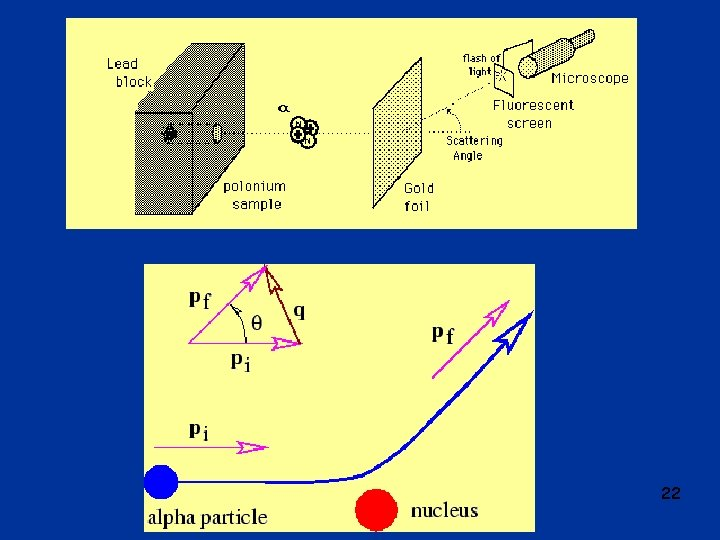22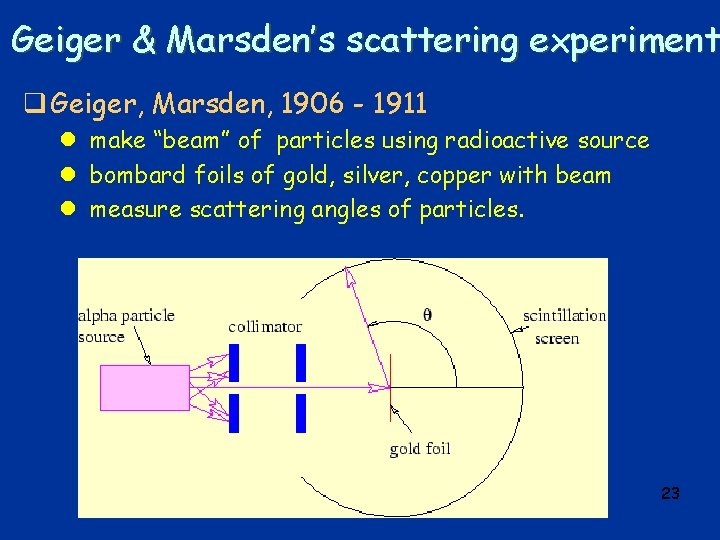Geiger & Marsden’s scattering experiment q Geiger, Marsden, 1906 - 1911 l make “beam” of particles using radioactive source l bombard foils of gold, silver, copper with beam l measure scattering angles of particles. 23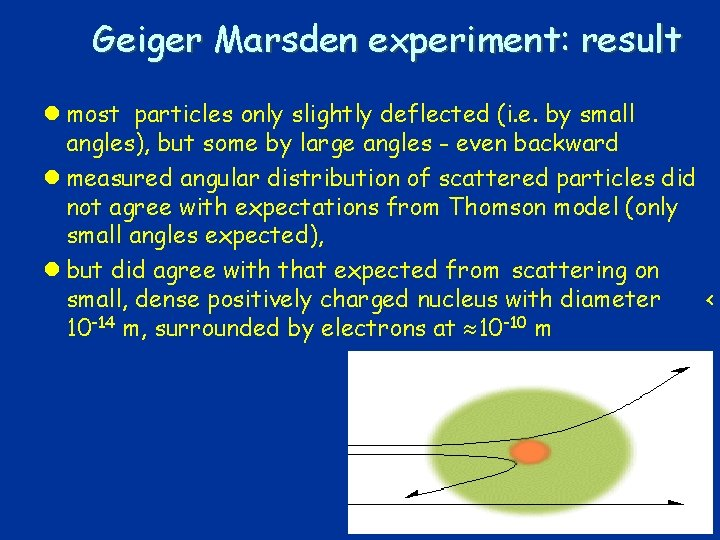Geiger Marsden experiment: result l most particles only slightly deflected (i. e. by small angles), but some by large angles - even backward l measured angular distribution of scattered particles did not agree with expectations from Thomson model (only small angles expected), l but did agree with that expected from scattering on small, dense positively charged nucleus with diameter < 10 -14 m, surrounded by electrons at 10 -10 m 24Rutherford model q “planetary model of atom” l positive charge concentrated in nucleus (<10 -14 m); l negative electrons in orbit around nucleus at distance 10 -10 m; l electrons bound to nucleus by electromagnetic force. 25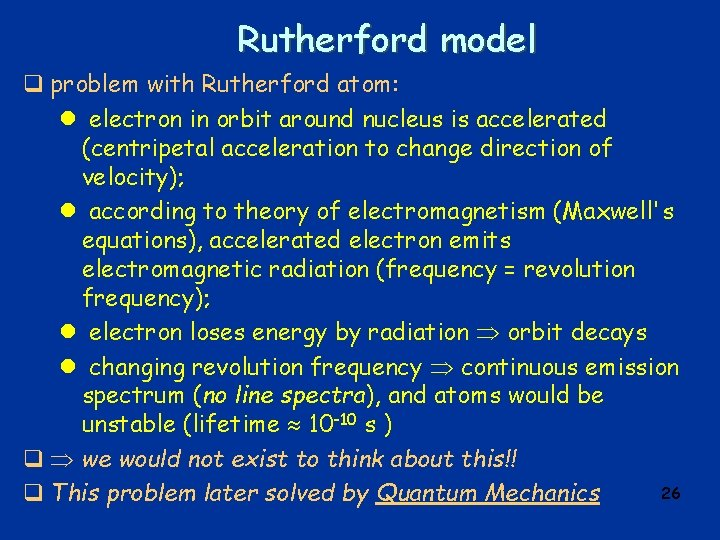Rutherford model q problem with Rutherford atom: l electron in orbit around nucleus is accelerated (centripetal acceleration to change direction of velocity); l according to theory of electromagnetism (Maxwell's equations), accelerated electron emits electromagnetic radiation (frequency = revolution frequency); l electron loses energy by radiation orbit decays l changing revolution frequency continuous emission spectrum (no line spectra), and atoms would be unstable (lifetime 10 -10 s ) q we would not exist to think about this!! 26 q This problem later solved by Quantum MechanicsBohr model of hydrogen (Niels Bohr, 1913) l Bohr model is radical modification of Rutherford model; discrete line spectrum attributed to “quantum effect”; l electron in orbit around nucleus, but not all orbits allowed; l three basic assumptions: o 1. angular momentum is quantized L = n·(h/2 ) = n ·ħ, n = 1, 2, 3, . . . electron can only be in discrete specific orbits with particular radii discrete energy levels o 2. electron does not radiate when in one of the allowed levels, or “states” o 3. radiation is only emitted when electron makes “transition” between states, transition also called “quantum jump” or “quantum leap” l from these assumptions, can calculate radii of allowed orbits and corresponding energy levels: l radii of allowed orbits: rn = a 1 · n 2 n = 1, 2, 3, …. , a 1 = 0. 53 x 10 -10 m = “Bohr radius” n = “principal quantum number” l allowed energy levels: En = - E 1 /n 2 , E 1 = “Rydberg energy” l note: energy is negative, indicating that electron is in a “potential well”; energy is = 0 at top of well, i. e. for n = , at infinite distance from the nucleus. 27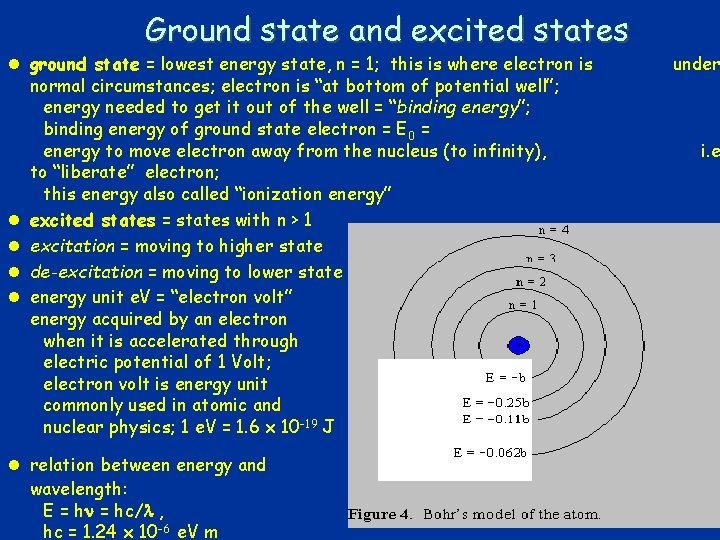Ground state and excited states l ground state = lowest energy state, n = 1; this is where electron is normal circumstances; electron is “at bottom of potential well”; energy needed to get it out of the well = “binding energy”; binding energy of ground state electron = E 0 = energy to move electron away from the nucleus (to infinity), to “liberate” electron; this energy also called “ionization energy” l excited states = states with n > 1 l excitation = moving to higher state l de-excitation = moving to lower state l energy unit e. V = “electron volt” energy acquired by an electron when it is accelerated through electric potential of 1 Volt; electron volt is energy unit commonly used in atomic and nuclear physics; 1 e. V = 1. 6 x 10 -19 J l relation between energy and wavelength: E = hc/ , hc = 1. 24 x 10 -6 e. V m under i. e = 28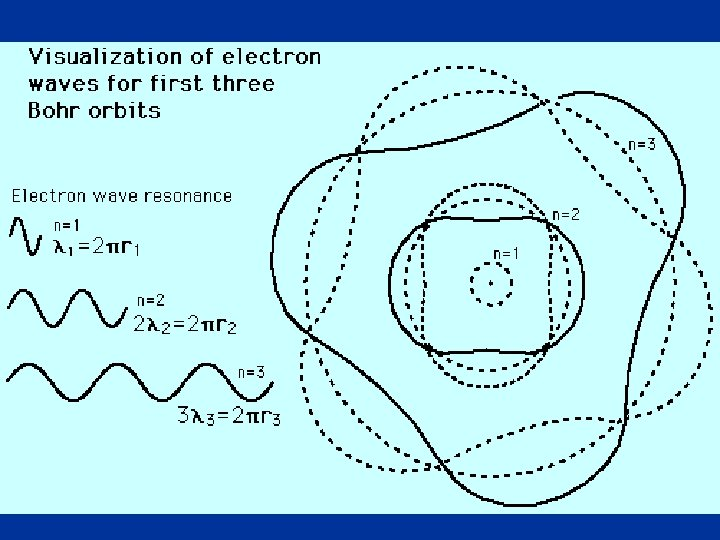29Excitation and de-excitation q PROCESSES FOR EXCITATION: l gain energy by collision with other atoms, molecules or stray electrons; kinetic energy of collision partners converted into internal energy of the atom; kinetic l energy comes from heating or discharge; l absorb passing photon of appropriate energy. q DE-EXCITATION: l spontaneous de-excitation with emission of photon which carries energy = difference of the two energy levels; l typically, lifetime of excited states is 10 -8 s (compare to revolution period 10 -16 s ) 30q Excitation: q states of electron in hydrogen atom: 31Energy levels and emission Spectra q En = E 1 · Z 2/n 2 q Hydrogen l En = - 13. 6 e. V/n 2 Lyman n=1 Balmer n=2 Paschen n=3 Balmer Series m=2 Visible UV 32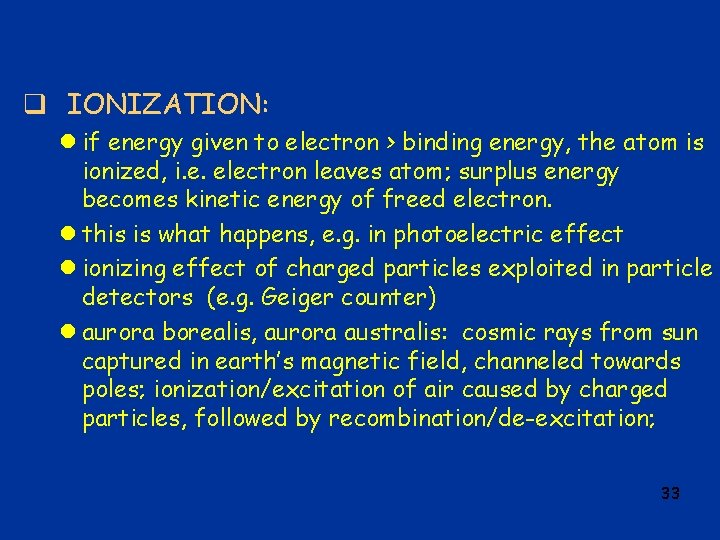q IONIZATION: l if energy given to electron > binding energy, the atom is ionized, i. e. electron leaves atom; surplus energy becomes kinetic energy of freed electron. l this is what happens, e. g. in photoelectric effect l ionizing effect of charged particles exploited in particle detectors (e. g. Geiger counter) l aurora borealis, aurora australis: cosmic rays from sun captured in earth’s magnetic field, channeled towards poles; ionization/excitation of air caused by charged particles, followed by recombination/de-excitation; 33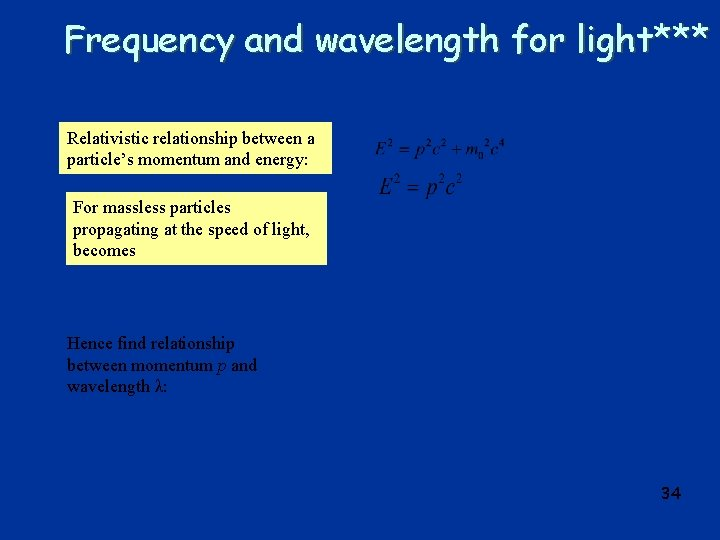Frequency and wavelength for light*** Relativistic relationship between a particle’s momentum and energy: For massless particles propagating at the speed of light, becomes Hence find relationship between momentum p and wavelength λ: 34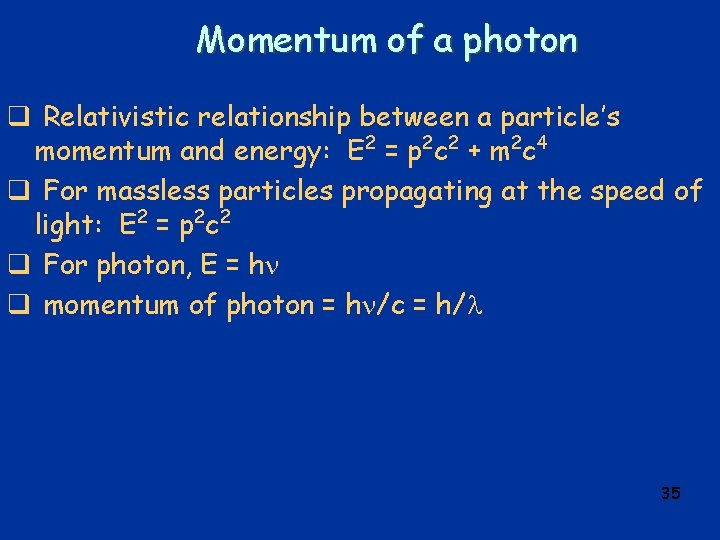Momentum of a photon q Relativistic relationship between a particle’s momentum and energy: E 2 = p 2 c 2 + m 2 c 4 q For massless particles propagating at the speed of light: E 2 = p 2 c 2 q For photon, E = h q momentum of photon = h /c = h/ 35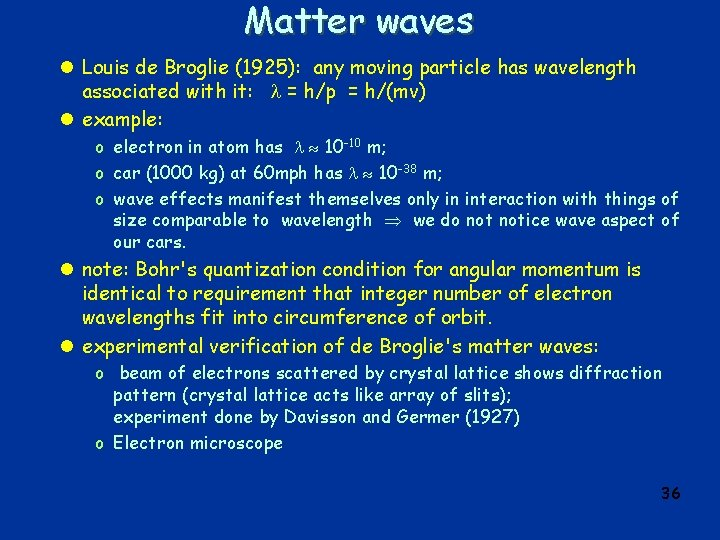Matter waves l Louis de Broglie (1925): any moving particle has wavelength associated with it: = h/p = h/(mv) l example: o electron in atom has 10 -10 m; o car (1000 kg) at 60 mph has 10 -38 m; o wave effects manifest themselves only in interaction with things of size comparable to wavelength we do notice wave aspect of our cars. l note: Bohr's quantization condition for angular momentum is identical to requirement that integer number of electron wavelengths fit into circumference of orbit. l experimental verification of de Broglie's matter waves: o beam of electrons scattered by crystal lattice shows diffraction pattern (crystal lattice acts like array of slits); experiment done by Davisson and Germer (1927) o Electron microscope 36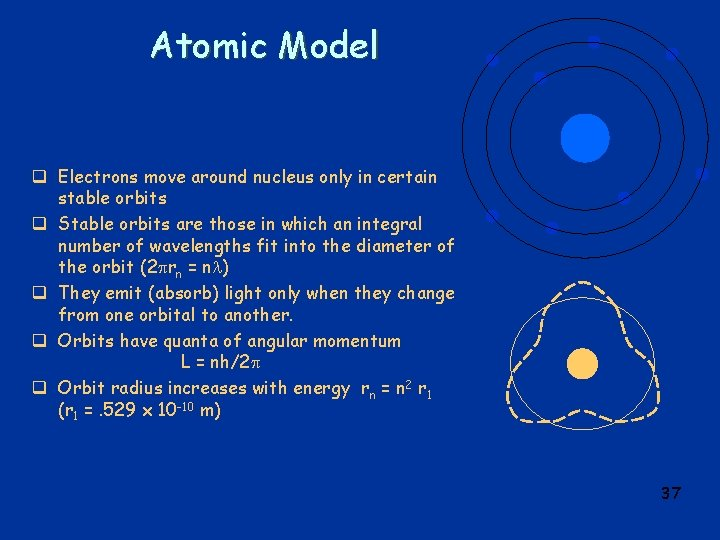Atomic Model q Electrons move around nucleus only in certain stable orbits q Stable orbits are those in which an integral number of wavelengths fit into the diameter of the orbit (2 rn = n ) q They emit (absorb) light only when they change from one orbital to another. q Orbits have quanta of angular momentum L = nh/2 q Orbit radius increases with energy rn = n 2 r 1 (r 1 =. 529 x 10 -10 m) 37QUANTUM MECHANICS q = new kind of physics based on synthesis of dual nature of waves and particles; developed in 1920's and 1930's. l Schrödinger equation: (Erwin Schrödinger, 1925) o is a differential equation for matter waves; basically a formulation of energy conservation. o its solution called “wave function”, usually denoted by ; o | (x)|2 gives the probability of finding the particle at x; o applied to the hydrogen atom, the Schrödinger equation gives the same energy levels as those obtained from the Bohr model; o the most probable orbits are those predicted by the Bohr model; o but probability instead of Newtonian certainty! l Uncertainty principle: (Werner Heisenberg, 1925) It is impossible to simultaneously know a particle's exact position and momentum (or velocity) p x ħ = h/(2 ) (remember h is a very small quantity: h = 6. 63 x 10 -34 J s = 4. 14 x 10 -15 e. V·s) (note that here p means “uncertainty” in our knowledge of the momentum p) l note that there are many such uncertainty relations in quantum mechanics, for any pair of “incompatible” observables. 38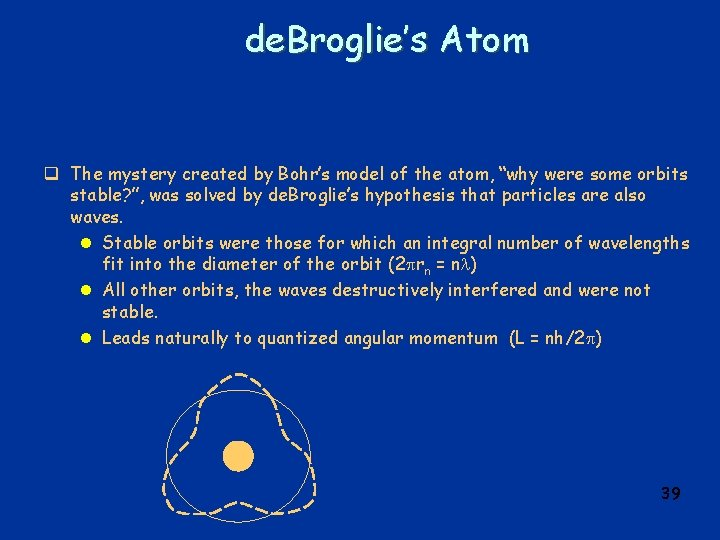de. Broglie’s Atom q The mystery created by Bohr’s model of the atom, “why were some orbits stable? ”, was solved by de. Broglie’s hypothesis that particles are also waves. l Stable orbits were those for which an integral number of wavelengths fit into the diameter of the orbit (2 rn = n ) l All other orbits, the waves destructively interfered and were not stable. l Leads naturally to quantized angular momentum (L = nh/2 ) 39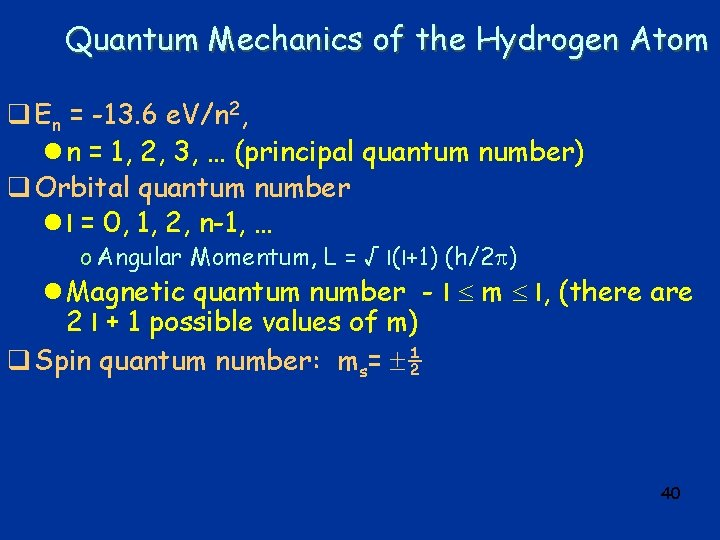Quantum Mechanics of the Hydrogen Atom q En = -13. 6 e. V/n 2, l n = 1, 2, 3, … (principal quantum number) q Orbital quantum number l l = 0, 1, 2, n-1, … o Angular Momentum, L = √ l(l+1) (h/2 ) l Magnetic quantum number - l m l, (there are 2 l + 1 possible values of m) q Spin quantum number: ms= ½ 40Multi-electron Atoms q Similar quantum numbers – but energies are different. q No two electron can have the same set of quantum numbers. q These two assumptions can be used to motivate (partially predict) the periodic table of the elements. 41Heisenberg Uncertainty Principle q Impossible to know both the position and the momentum of a particle precisely. l A restriction (or measurement) of one, affects the other. l x p h/(2 ) q Similar constraints apply to energy and time. l E t h/(2 ) EXAMPLE: If an electron's position can be measured to an accuracy of 1. 96× 10 -8 m, how accurately can its momentum be known? x p h/(2 ) p = h/(2 x) p = 6. 63 x 10 -34 Js /(2 1. 96 x 10 -8 m) = 5. 38 x 10 -27 N s 42Periodic table q Exclusion Principle: l No two electrons in an atom can occupy the same quantum state. q When there are many electrons in an atom, the electrons fill the lowest energy states first: l lowest n l lowest l l lowest ms q this determines the electronic structure of atoms 43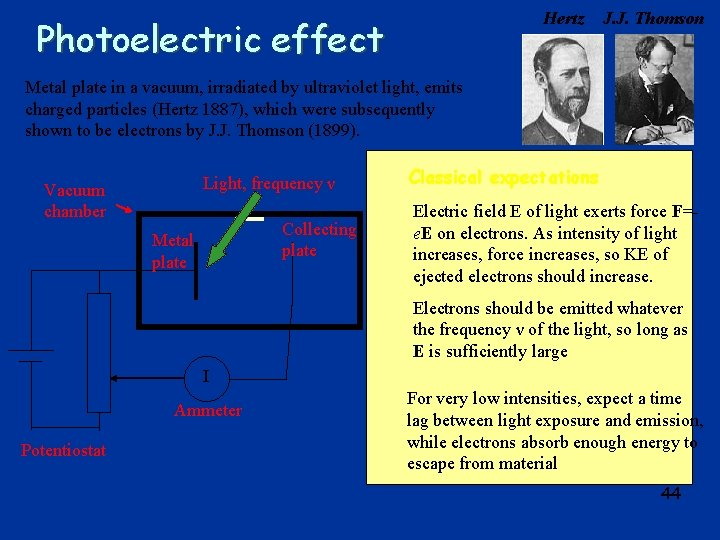Hertz Photoelectric effect J. J. Thomson Metal plate in a vacuum, irradiated by ultraviolet light, emits charged particles (Hertz 1887), which were subsequently shown to be electrons by J. J. Thomson (1899). Light, frequency ν Vacuum chamber Collecting plate Metal plate Classical expectations Electric field E of light exerts force F=e. E on electrons. As intensity of light increases, force increases, so KE of ejected electrons should increase. Electrons should be emitted whatever the frequency ν of the light, so long as E is sufficiently large I Ammeter Potentiostat For very low intensities, expect a time lag between light exposure and emission, while electrons absorb enough energy to escape from material 44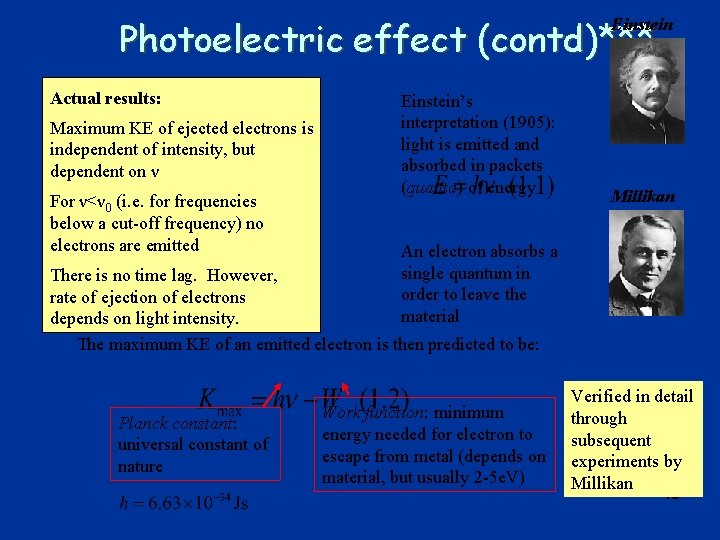Photoelectric effect (contd)*** Einstein Actual results: Maximum KE of ejected electrons is independent of intensity, but dependent on ν For ν<ν 0 (i. e. for frequencies below a cut-off frequency) no electrons are emitted Einstein’s interpretation (1905): light is emitted and absorbed in packets (quanta) of energy Millikan An electron absorbs a single quantum in order to leave the material There is no time lag. However, rate of ejection of electrons depends on light intensity. The maximum KE of an emitted electron is then predicted to be: Planck constant: universal constant of nature Work function: minimum energy needed for electron to escape from metal (depends on material, but usually 2 -5 e. V) Verified in detail through subsequent experiments by Millikan 45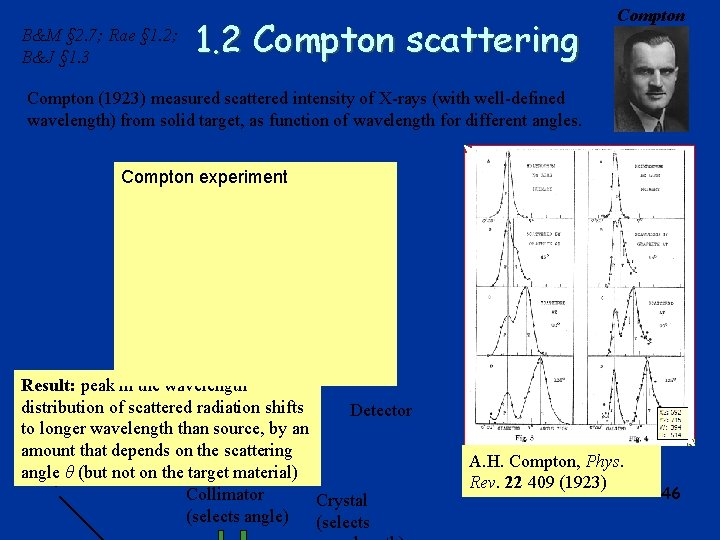B&M § 2. 7; Rae § 1. 2; B&J § 1. 3 1. 2 Compton scattering Compton (1923) measured scattered intensity of X-rays (with well-defined wavelength) from solid target, as function of wavelength for different angles. Compton experiment Result: peak in the wavelength distribution of scattered radiation shifts Detector to longer wavelength than source, by an amount that depends on the scattering angle (but not on the target material) X-ray θsource Collimator Crystal (selects angle) (selects A. H. Compton, Phys. Rev. 22 409 (1923) 46Compton scattering (contd) Classical picture: oscillating electromagnetic field would cause oscillations in positions of charged particles, re-radiation in all directions at same frequency and wavelength as incident radiation Before Photon Incoming photon p After θ Electron Compton’s explanation: “billiard ball” collisions between X-ray photons and electrons in the material Conservation of energy: p’ φ pe Conservation of momentum: 47Compton scattering (contd) Assuming photon momentum related to wavelength: ‘Compton wavelength’ of electron (0. 0243 Å) 48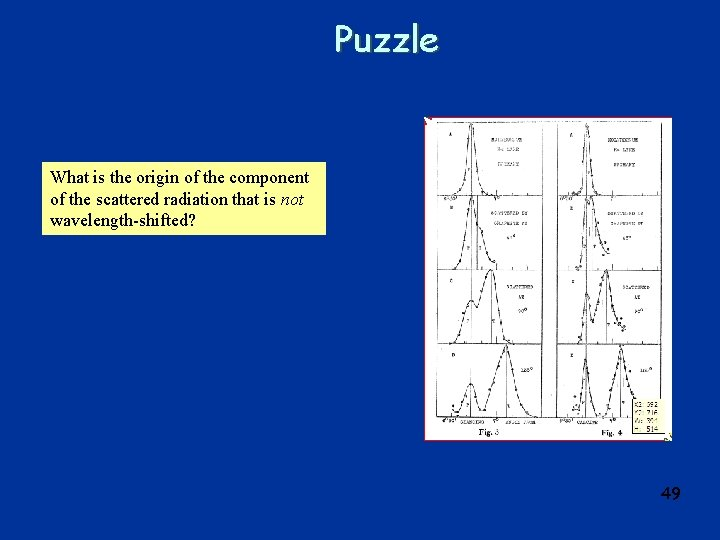Puzzle What is the origin of the component of the scattered radiation that is not wavelength-shifted? 49Wave-particle duality for light*** “ There are therefore now two theories of light, both indispensable, and - as one must admit today despite twenty years of tremendous effort on the part of theoretical physicists - without any logical connection. ” A. Einstein (1924) • Light exhibits diffraction and interference phenomena that are only explicable in terms of wave properties • Light is always detected as packets (photons); if we look, we never observe half a photon • Number of photons proportional to energy density (i. e. to square of electromagnetic field strength) 50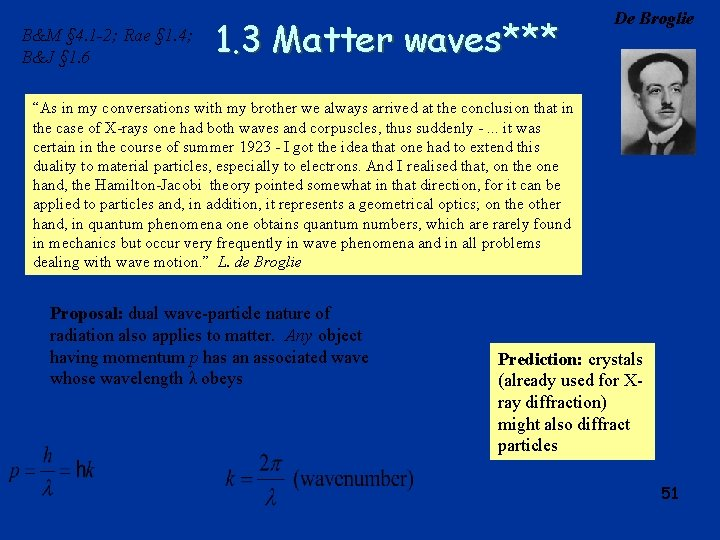B&M § 4. 1 -2; Rae § 1. 4; B&J § 1. 6 1. 3 Matter waves*** De Broglie “As in my conversations with my brother we always arrived at the conclusion that in the case of X-rays one had both waves and corpuscles, thus suddenly -. . . it was certain in the course of summer 1923 - I got the idea that one had to extend this duality to material particles, especially to electrons. And I realised that, on the one hand, the Hamilton-Jacobi theory pointed somewhat in that direction, for it can be applied to particles and, in addition, it represents a geometrical optics; on the other hand, in quantum phenomena one obtains quantum numbers, which are rarely found in mechanics but occur very frequently in wave phenomena and in all problems dealing with wave motion. ” L. de Broglie Proposal: dual wave-particle nature of radiation also applies to matter. Any object having momentum p has an associated wave whose wavelength λ obeys Prediction: crystals (already used for Xray diffraction) might also diffract particles 51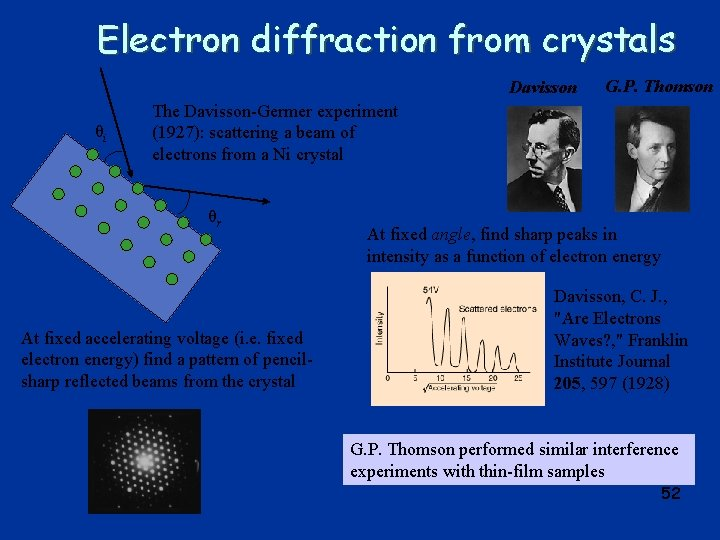Electron diffraction from crystals Davisson θi G. P. Thomson The Davisson-Germer experiment (1927): scattering a beam of electrons from a Ni crystal θr At fixed accelerating voltage (i. e. fixed electron energy) find a pattern of pencilsharp reflected beams from the crystal At fixed angle, find sharp peaks in intensity as a function of electron energy Davisson, C. J. , "Are Electrons Waves? , " Franklin Institute Journal 205, 597 (1928) G. P. Thomson performed similar interference experiments with thin-film samples 52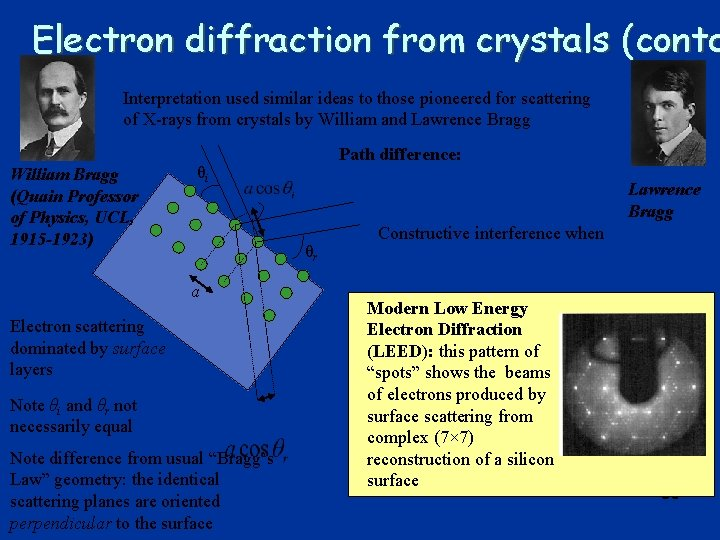Electron diffraction from crystals (contd Interpretation used similar ideas to those pioneered for scattering of X-rays from crystals by William and Lawrence Bragg William Bragg (Quain Professor of Physics, UCL, 1915 -1923) Path difference: θi Lawrence Bragg θr a Electron scattering dominated by surface layers Note θi and θr not necessarily equal Note difference from usual “Bragg’s Law” geometry: the identical scattering planes are oriented perpendicular to the surface Constructive interference when Modern Low Energy Electron Diffraction (LEED): this pattern of “spots” shows the beams of electrons produced by surface scattering from complex (7× 7) reconstruction of a silicon surface 53The double-slit interference experiment Originally performed by Young (1801) with light. Subsequently also performed with many types of matter particle (see references). y d θ Incoming beam of particles (or light) D Detecting screen (scintillators or particle detectors) Alternative method of detection: scan a detector across the plane and record arrivals at each point 54Results Neutrons, A Zeilinger et al. 1988 Reviews of Modern Physics 60 1067 -1073 He atoms: O Carnal and J Mlynek 1991 Physical Review Letters 66 2689 -2692 Fringe visibility decreases as molecules are heated. L. Hackermüller et al. 2004 Nature 427 711 -714 C 60 molecules: M Arndt et al. 1999 Nature 401 680682 With multiple -slit grating Without grating 55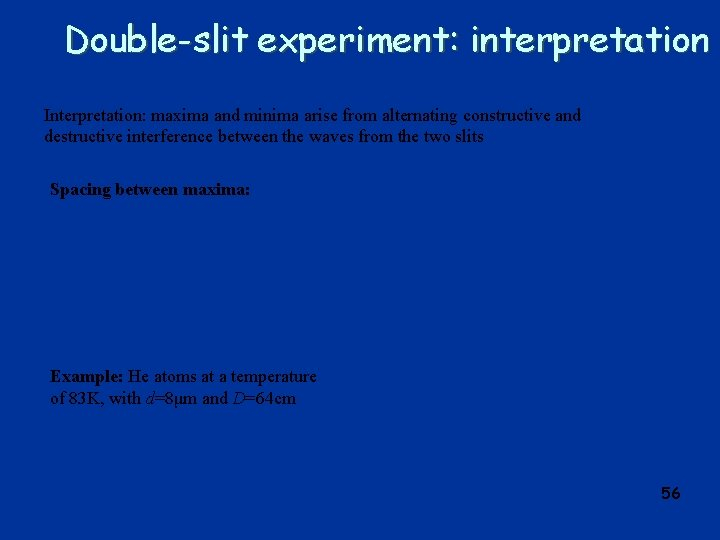Double-slit experiment: interpretation Interpretation: maxima and minima arise from alternating constructive and destructive interference between the waves from the two slits Spacing between maxima: Example: He atoms at a temperature of 83 K, with d=8μm and D=64 cm 56Double-slit experiment: bibliography 57Matter waves: key points*** q Interference occurs even when only a single particle (e. g. photon or electron) in apparatus, so wave is a property of a single particle l A particle can “interfere with itself” q Wavelength unconnected with internal lengthscales of object, determined by momentum q Attempt to find out which slit particle moves through causes collapse of interference pattern (see later…) Wave-particle duality for matter particles • Particles exhibit diffraction and interference phenomena that are only explicable in terms of wave properties • Particles always detected individually; if we look, we never observe half an electron • Number of particles proportional to…. ? ? ? 58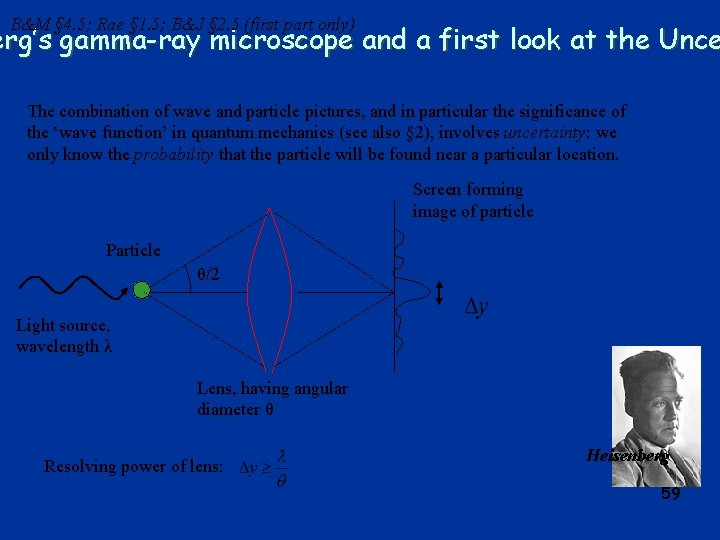B&M § 4. 5; Rae § 1. 5; B&J § 2. 5 (first part only) erg’s gamma-ray microscope and a first look at the Unce The combination of wave and particle pictures, and in particular the significance of the ‘wave function’ in quantum mechanics (see also § 2), involves uncertainty: we only know the probability that the particle will be found near a particular location. Screen forming image of particle Particle θ/2 Light source, wavelength λ Lens, having angular diameter θ Resolving power of lens: Heisenberg 59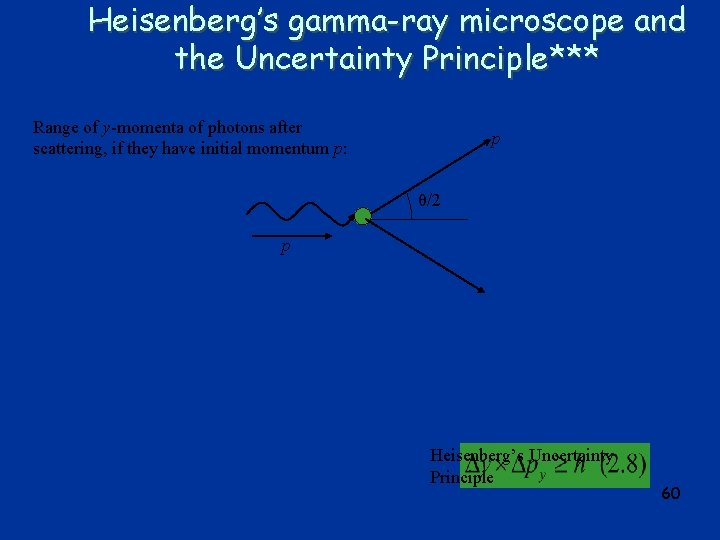Heisenberg’s gamma-ray microscope and the Uncertainty Principle*** Range of y-momenta of photons after scattering, if they have initial momentum p: p θ/2 p Heisenberg’s Uncertainty Principle 60